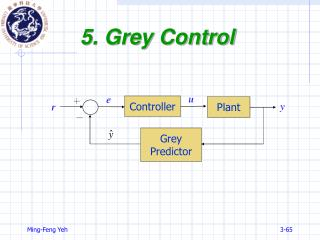DownloadDownload Presentation5. Grey Control

# 5. Grey Control

Download Presentation## 5. Grey Control

- - - - - - - - - - - - - - - - - - - - - - - - - - - E N D - - - - - - - - - - - - - - - - - - - - - - - - - - -
##### Presentation Transcript

1. u e ＋ y r 5. Grey Control Controller Plant － Grey Predictor

2. Grey Prediction Control • The control principle of control theory, whether classical or modern, is to control system behavior according to the state sample which has already occurred. • The demerits of the afterward manner are • Impossible to avert accidents in advance • Impossible to control timely • Weakly adaptable

3. Essential Idea • The essential idea is to control the system behavior in advance with the control strategy obtained from the prediction controller based on GM(1,1) model. • Maintain a desired state within reasonably accurate tolerances even though the output is varied. Reference Signal Control Signal Output Signal Plant Decision-Making Mechanism r u y Predictive Signal Grey Predictor

4. GM(1,1) Models • x(0) = {x(0)(1), x(0)(2),…, x(0)(n)}, data length = n • Whole data GM(1,1) model: data length = n • Partial data GM(1,1) model: 4 ≦data length < n • New Information GM(1,1) model: add the newest datum x(0)(n+1) to the original series x(0) , i.e., {x(0)(1), x(0)(2),…, x(0)(n), x(0)(n+1)} • Equal-dimensionalNew Information GM(1,1) model: delete the oldest datum and add the newest one, i.e., {x(0)(2), x(0)(3),…, x(0)(n), x(0)(n+1)}

5. Example 5.1

6. Rolling Prediction • x= {x(1), x(2),…, x(n)}, data length = n • Step 1: Construct the m-data series x(0) (m<n) from the considered series x. • Step 2: Measure the predictive values by the GM(1,1) modelbuilt by the m-data series x(0). • Step 3: Update the m-data series bydeleting the oldest datum and adding the newest one, and then return to Step 2.

7. Example 5.2

8. Example 5.3-(1) • Data length: m=8 • One-step-aheadprediction • Error: 6.83%

9. Example 5.3-(2) • Data length: m=4 • One-step-aheadprediction • Error: 2.17%

10. Grey Prediction Control Step 1: Construct the m-data input series from the sensitized signal Step 2: Forecast the p-step-ahead predictive value of y(k) by grey predictor Step 3: Decision-making Step Step 4: Update the m-data input series and then go to Step 2 u e ＋ Controller Plant r y － Grey Predictor Adjust step-size

11. Example 5.4 • Transfer function of plant: • Transfer function of controller: • Time interval: • Sampling interval: 0.05 sec. • Reference signal:

12. Example 5.4-(1) • Data length: m = 4 • Predictive step size = 1 • Reference signal red line • With predictor black line • Without predictor blue line

13. Example 5.4-(2) • Data length: m = 4 • Predictive step size = 2 • Reference signal red line • With predictor black line • Without predictor blue line

14. Example 5.4-(3) • Data length: m = 4 • Predictive step size = 1 • Reference signal red line • With predictor black line • Without predictor blue line